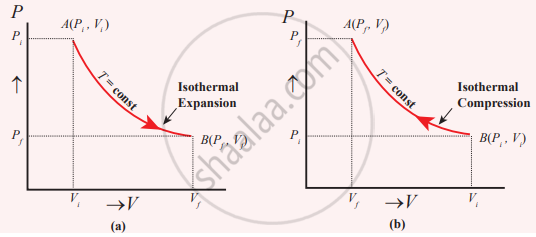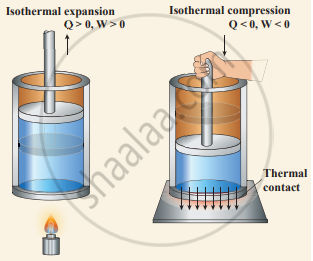Tamil Nadu Board of Secondary EducationHSC Science Class 11th

# Explain in detail the isothermal process. - Physics

Explain in detail the isothermal process.

#### Solution

Isothermal process: It is a process in which the temperature remains constant but the pressure and volume of a thermodynamic system will change. The ideal gas equation is
PV = µRT

Here, T is constant for this process So the equation of state for the isothermal process is given by

PV = Constant ..................…(1)

This implies that if the gas goes from one equilibrium state (P1, V1) to another equilibrium state (P2, V2) the following relation holds for this process

P1V1 = P2V2 ….............(2)

Since PV = constant, P is inversely proportional to "V" ("P" ∝ 1/"V"). This implies that the PV graph is a hyperbola. The pressure-volume graph for constant temperature is also called isotherm. We know that for an ideal gas the internal energy is a function of temperature only. For an isothermal process since the temperature is constant, the internal energy is also constant. This implies that dU or ∆U = 0.

For an isothermal process, the first law of thermodynamics can be written as,

Q = W …...........(3)(a) Quasi-static isothermal expansion (b) Quasi-static isothermal compressionIsothermal expansion and isothermal compression

From equation (3), we infer that the heat supplied to gas is used to do only external work. It is a common misconception that when there is a flow of heat to the system, the temperature will increase. For the isothermal process, this is not true. The isothermal compression takes place when the piston of the cylinder is pushed. This will increase the internal energy which will flow out of the system through thermal contact.

Concept: Thermodynamic Process
Is there an error in this question or solution?
Chapter 8: Heat and Thermodynamics - Evaluation [Page 159]

#### APPEARS IN

Tamil Nadu Board Samacheer Kalvi Class 11th Physics Volume 1 and 2 Answers Guide
Chapter 8 Heat and Thermodynamics
Evaluation | Q III. 13. | Page 159
Share